# Why can’t you divide by zero?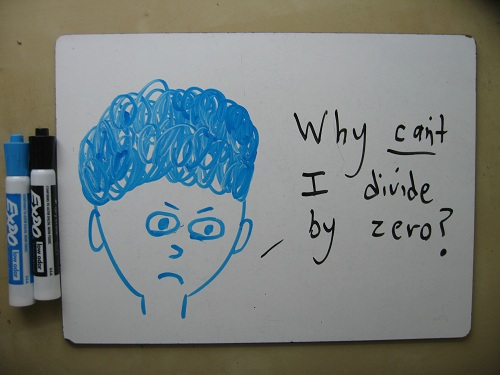Part 1: Dividing By Smaller and Smaller Numbers
by a High School Math TeacherSuppose you’ve got a pizza. A nice charcoal-cooked one from New Haven, or an oven-hot Chicago deep-dish, or even one of those organic San Francisco artisan pies that somehow make artichoke hearts seem like they belong on a pizza. And, generous soul that you are, you’ve decided to share.

How many people can you feed if everybody gets half of a pizza (a hearty helping)?

Well, it’s 1 pizza ÷ ½ pizzas per person = 2 people.And how many can you feed if everybody gets 1/10 of a pizza (a cheesy snack)?

1 pizza ÷ 0.1 pizzas per person = 10 people.And how many if everybody gets 1/100 of a pizza (a bite-sized morsel)?

1 pizza ÷ 0.01 pizzas per person = 100 people.And how many can you feed if each gets 1/1000 of a pizza (a crumb with a dab of sauce)?

1 pizza ÷ 0.001 pizzas per person = 1000 people.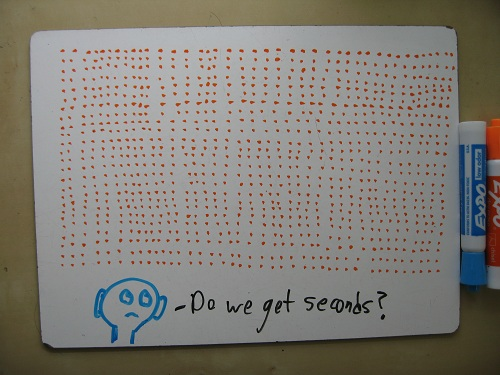The smaller the slice you give to each person, the more people you can feed. Or, more abstractly: the smaller the number you divide by, the bigger the result.

Now, take it one step further: What if each person gets 0% of a pizza?

1 pizza ÷ 0 pizzas per person = ???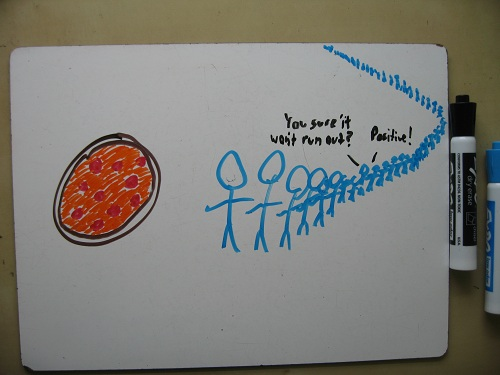How many people can you feed? Well, there’s no limit, because you’re not actually feeding them anything. If the earth’s seven billion people all show up at your door, asking for their share of pizza, you can say “No problem!” because “their share of pizza” amounts to nothing at all. Add another seven billion, and you’d say the same thing. How many people can you feed? There’s no answer.

When you divide a number by 0, there’s no single answer. To divide is to break something into piles of a certain size. And breaking something into piles of size zero just doesn’t make sense.

Part 2: “Inverse of Multiplication”
by a Math PhD Candidate

While she was washing dishes, I asked my fiancee why you can’t divide by zero. Her off-the-top-of-her-head answer was more concise than mine. (In my defense, I get the dishes cleaner than she does.)

When you divide by a number – let’s say 4 – you’re asking, “How many times can 4 go into the number?” So: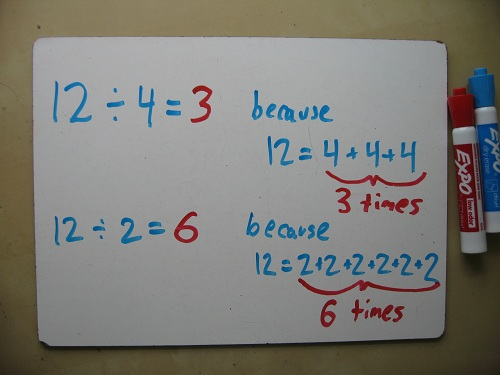But when you divide by 0, you’re asking, “How many times can 0 go into the number?” And no matter how many zeroes you add, 0 + 0 + 0 + 0 … will never equal 12. So 12 ÷ 0 is undefined.

Part 3: “Inverse of Multiplication” Redux
by an Elementary-Level Math Specialist

I then ran both of these explanations by my sister Jenna, a K-8 math specialist. She liked Taryn’s answer, and gave her own even more concise version.

Division is the inverse of multiplication. So when you divide 12 by 4, you’re saying: “What times 4 gives you 12?”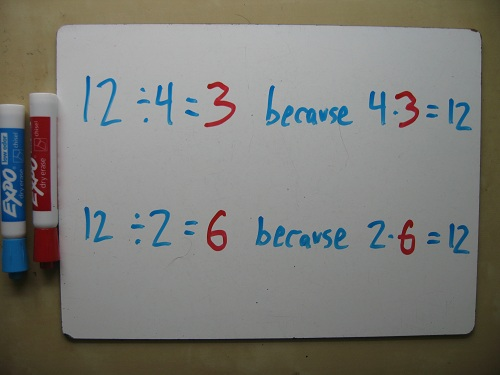Therefore, dividing by zero is like asking, “What times 0 gives you 12?” There’s obviously no answer, since any multiple of 0 will be 0.Part 4: Tying it All Together

At dinner with my father James (a professor of Operations Research), I asked him to explain why you can’t divide by zero. He gave an explanation pretty similar to mine, and then summarized the relative merits of the two approaches quite nicely.

The Taryn/Jenna explanation, he said, cuts to the chase, and will satisfy a wider (and younger) audience. It starts by saying, “Well, here’s what division is,” and then showing that the concept makes no sense when applied to zero.

The Ben/James explanation, meanwhile, is valuable because it doesn’t cut to the chase. It connects the question “Can you divide by zero?” to other ideas (limits and asymptotic behavior), and gets more to the conceptual heart of the problem.

Anyway, there you have it. Four math professionals, two basic explanations, and one more blog adding its voice to the din of answers on this subject.

## 67 thoughts on “Why can’t you divide by zero?”

1.Farid says:

In High School my brother told me: let’s say A=1 and B=2 , therefor we car write : Ax0=0 and Bx0=0
then Ax0=Bx0 => Ax(0/0)= Bx(0/0) and we get A=B, so 1=2. 0/0 is legit

1.Ben Orlin says:

Mmm, good point. I’ve always suspected 1 = 2.

1.Mark Ross says:

This is only true for very large values of 1, or very small values of 2.

2.mrdardy says:

Ben

My answer is pretty similar to part three except when I write the example, I say “Let’s assume that 12 / 0 = some number, let’s call it N. Then when we transform the equation as 12 = N * 0 and I ask them whether this is ever true, I get a chorus of “NO” I then underline the N * 0 and point out that we spelled NO.” Conversation concludes with a chorus of groans but I have had students report that they remember the conversation. The key takeaway is simply creating some space so that the question WHY gets asked and answered.

1.Ben Orlin says:

Ooh, I like that. “Chorus of groans” is how you know you’re doing something right.

1.Adam says:

Agreed. That’s up there with “writing ‘sin(x)’ without the angle is a sin!”

1.Ben Orlin says:

Seriously, though – a mortal sin!

2.Mary Rose Beeken says:

Why can’t there be a number that means both negative and positive infinity? That number could be the answer to our divide by zero problem. Of course there isn’t so someone should come up with it.

3.samiJ says:

I go with the Billy Preston explaination.

1.Ben Orlin says:

Not totally convinced by the mathematics of it, but… ah, who am I kidding, of course I am.

4.Geoff says:

Good call on linking to Modern–clearly the best in New Haven.

1.Ben Orlin says:

So true. I get where the Sally’s and Pepe’s people are coming from. But Modern is Modern.

5.Creative Metaphor says:

Even though they are, I guess I never think of fractions as division, but as ratios (and then thanks to engineering, decimals). 1/2 – Out of two, I have one. 5/12 – Out of twelve, I have five. 0/3 – Out of three, I have zero. 2/0 – Out of zero, I have… two? Arguably, quantum physics and the Federal Reserve might agree with that, but as far as most of us are concerned, I can’t have two of something if there are zero things to be had!
🙂

1.Ben Orlin says:

Mmm, I like that way of thinking about it. (And the US treasury must LOVE that way…)

6.Taylor Buscher says:

It’s true that dividing by zero never makes sense, but it can be a fun mindbending thought experiment to think of what it would be like if x/0 really did have meaning for any given x…you get a whole new kind of number.

1.Ben Orlin says:

One of my students did a similar thing with 0.99999… He really wanted it to be a number just a little bit smaller than 1. To do that, he had to define a number epsilon, with all the same properties as zero, but which isn’t zero. Playing around like that is a fun way to explore the number system!

1.Creative Metaphor says:

O.O
OMG that’s really kinda awesome!

7.Gord Goebel says:

I’ve always thought of it this way:
Multiplication is just a fancy (repetitive) kind of addition. “What do you get if you add six three times?”
Division is a fancy kind of subtraction. “How many times can you take two from twelve before it’s all gone?”
So “How many times can you take zero away from twelve before it’s all gone?” F’rever.

1.Ben Orlin says:

Elegant – I like that.

2.Scot says:

This is honestly true. Divide by zero is a problem computers are unable to computer because it is unable to handle infinity.
x/0 simply means it was never divided. That has two possible answers. Either the answer is 1 or infinity depending on your point of view, and I think the solution is to make it a given in the problem.

i.e. given: divide by zero = 1 or
given divide by zero = infiniity

1.DontAskWontTell says:

Well it never divides. As the original comment states, how many times can you take away until nothing remains. infinity doesn’t suffice because you will never be left with anything less than what is. It should be a totally new concept (which it is). You could give the inability to divide by zero a symbol (æ?) but it is essentially still referring to the inability to divide by zero.

8.Evan says:

Thank God! This finally makes sense! Thank you for bad drawings of pizza.

9.Saburou Saitoh says:

By a very natural extension of fractions, we will be able to obtain a very beautiful simple result. Please look the attached paper.
M. Kuroda, H. Michiwaki, S. Saitoh, and M. Yamane,
New meanings of the division by zero and interpretations on \$100/0=0\$ and on \$0/0=0\$,
Int. J. Appl. Math. Vol. 27, No 2 (2014), pp. 191-198, DOI: 10.12732/ijam.v27i2.9.

S. Saitoh, Generalized inversions of Hadamard and tensor products for matrices, Advances in Linear Algebra \& Matrix Theory. Vol.4 No.2 2014 (2014), 87-95. http://www.scirp.org/journal/ALAMT/

1.DontAskWontTell says:

This assumes that the result means the amount for each holding. This is an incorrect view of division. Division is more concisely defined in the above comments as being how many times you can take away until nothing is left. Results based on wrong information are always wrong, whether it be literally or in intention. In this case it’s literally wrong.

10.ChocolateyBuddy says:

The concept of dividing by 0 makes sense. I like how he used the pictures to portray what he meant. 6×2 does indeed create 12. 12/2 does indubitably reduce to 6. 12 truly cannot be split up into 0 parts because it does not make sense.

11.Kimberly Urbeck says:

I have always been taught that nothing times nothing is nothing. Likewise with dividing any number divided by zero is zero.

12.Sam says:

And the answer to the whole “why isn’t it just infinity” thing: It can be. There are perfectly legitimate, consistent mathematical systems in which 1/0 = infinity (but 0/0 is still a mess). You just lose negative numbers (and thus lose a whole lot of subtractions in return for a few extra divisions). The reason you can’t have negative numbers is that as you get closer from the negative side, you approach minus infinity, and from the positive side, you get plus infinity. We like having our operations nice and continuous, so we’re stuck.

13.Ian Montgomery says:

The way I see it is -try saying it in straight English. If you divide something by nothing what is the answer. The answer is you haven’t done anything. So it is undefined.

1.Evan Drury says:

But then by that same logic if you multiply something by nothing the answer is also that you haven’t done anything. However it is defined; as zero.

14.The boy who cried shut up says:

Next time I get my maths question wrong. Divides by zero. World ends.

15.MainFragger says:

The thing I’d love to be able to do someday is make a slight conception differentiation between what it means to divide by “1” and “0” as they are currently explained. For me, if you divide by one, you are actually considering the math of how many times a single slice fits into a pizza..but if you divide by “0” you are really saying, “eh..I don’t really care how many slices can be in this pizza, as long as I have the pizza..” You can technically then eat the pizza whole.

But the other reason i like the idea of being able to divide by zero is to represent a neutral process that occurred..maybe it was witnessable, maybe not, but either way, it happened, shifted from 0, but then something else happened and shifted it back to 0.. so that by the time the math problem works it self out..its kind of like someone pulled the table cloth out from everything on the table without anything moving, and then put it back again, without anything moving. Somethng happened, but there is no apparent perceivable change in status of the pizza pie..or whatever you were doing…

1.gordiegii says:

The problem with magicians is that you can never be sure what you’ll end up with. If they pull the tablecloth out from under a pizza, it might spontaneously turn into a rabbit.

16.MainFragger says:

My counter to the 15X0= 0+0+0+0..etc is that you have to understand that adding zero to something is actually NOT doing something or NOT adding, multiply…so to me, when you say 15X0, you are saying that “I have 15, and I am not adding or subtracting anything to it, so I in effect, still have 15″…on the other hand, 0X15 is 0, because you are starting with 0 and adding 0 to it 15 times.

1.gordiegii says:

So by your logic if 15×0 is 15 then 15 / 0 is also 15. However that sounds like 1, ie 15×1 = 15 and 15 / 1 = 15, thefore 0=1

1.MainFragger says:

When you divide something by 1 you have x many of those pieces. When you divide by 0, you the whole of the item undivided that contains as many pieces (or the same amount of material) as if you had divided by 1. If you want to make your 0=1 to make sense, it should read 0=1 where x is divided by either number .

2.gordiegii says:

I guess my point is that with the addition analogy is that with 15 x 3 you are not adding 15 to itself 3 times (that would give you 60) but adding 15 to “the result” 3 times. When you start “the result” is zero. With division it is subtraction multiple times: how many times can I take away 15 from 45 until there’s 0 left? 3 times.
So how many times can you take 0 from 15 before there’s 0 zero left? 0? No. 1? No. Infinity? No there’s no number of times you can take 0 away from 15 to have 0 left.

1.MainFragger says:

But the thing is, just because math works that way on most items, doesn’t mean we should ALWAYS expect it to work that way. In this case, counting down to 0 is irrelevant, because by dividing by 0 you are technically NOT dividing, and therefor would never get to 0, in point of fact, if dividing x by 0, you should always end up with x.

2.MainFragger says:

I realize its taken me a long time to add to this, buuuut.. There is a bit of a language guffaw that occurs when you multiply by something. 15×3 can actually be thought of in two ways. You can argue that you are multiplying 15 three times and its 0+15+15+15…or you could say that you have three times the amount of fifteen..which is 15+(15+15)(but you have to understand that the numbers of +x that exist in the parenthesis are depending on how many time you would normally add a number to itself to get the amount of items resultant from the number of times you are multiplying by). Its a weird distinction but depending on what problem you are trying to solve over all, its one that is occasionally worth making.

1.MainFragger says:

just briefly expand on that..

10X5..We know that is going to equal 50…
So, how does the math shake out?

10+(10(does this equal 50..no, then..)+10(does this equal 50..no, then..)+10(does this equal 50..no, then..)+10(does this equal 50..yes, then..)=50..

3.DontAskWontTell says:

This started from a ridiculous statement, as in my other replies, results based on wrong information are always wrong.
You never had fifteen dude, hence the zero. you have no fifteens. how much do you have based on having no fifteens? The answer is nothing. You have nothing. nothing = 0

1.DontAskWontTell says:

To expand on that
15×0 = (there are no places to put a 15)
0x15 = (0+0+0+0+0+0+0+0+0+0+0+0+0+0+0)
(^ there are 15 places to put a 0)

1.MainFragger says:

I don’t have 15 of what? Maybe I do? I dunno..why did you bring up 15 to multiply it by 0 if no one had it to begin with? While we on this subject, have you seen the sweat stain on that guy’s shirt that looks like Elvis? Whats that got to do with anything? I don’t know..ask 15..

2.MainFragger says:

Ok..so let me address this in a slightly less condescending and smarta** way…

I have and always will think of the left number of a multiplication as something you already have and the right side of the multiplication is what you are going to multiply that amount by.. If you didn’t have 15 to begin with…why would bother to worry about multiplying it by 0 to begin with. That makes no sense. (I have nothing, which may or may not be 15..I’m not really sure..heck..lets multiply that by 0 and see what happens…you must be really really bored.) I am not a computer. I don’t need a memory space to put a number into in order to get correct result. I just need to know that the number should be there. When you put 15X0, I read it as “I have 15 of something and I am gonna multiply that by 0” At least at first, over time, my real evaluation of that formula is, “I have 15 of something, and I’m going to go through the motions of multiplying it by 0, which is really not gonna multiply it by anything”.

Still kind of silly, but at least it provides a real world answer if I want one.

17.Evan Drury says:

Really, you have to think of this in equal terms. This whole “divide by zero” concept seem alien because on the outside it seems like an exception to all the math rules. However, there is actually an opposite to this problem. As was illustrated, you can divide by 0.01, 0.00000001, and even -0.0000000000001 because they are all measurable values that will give a measurable result. However, as the decimal places increase, so too does the number of decimal places in the answer (even if they are trailing zeros, they must still be calculated). When dividing by zero, it becomes basically a situation where the number of decimal places are so infinitely long that it cannot be computed as it never ends. Basically a repeating zero decimal place.

So, if you’re still with me, the opposite of this equation then is multiplying anything by infinity. Once again, this too would be undefined, “ERROR”, or however you want to look at it. Like this, the rule holds: You can’t divide by nothing and you can’t multiply by everything.

18.fiddlestix says:

well, there is no such thing as zero in existence, as a concept it wasn’t invented until quite a lot later on in the development of mathematics. If you divide by zero, what you are actually stating is that, ‘I do not want to divide this thing at all’ and so ‘I want to return the thing undivided’. Therefore divide by zero is actually another equality operator. This makes sense for a number of reasons. The first is that any number can be shown to be itself + 1 – 1.9999 recurring(infinity) is the same as 2, or to put another way, since mathematics and accruement of stuff is so well equated, when I have two apples, I am on to my third (1999 is the 2000th year).
That infinity is equivalent to 1 and 0 can be demonstrated geometrically in the following way. Take a multiple sided dice like one might find in an advanced board game – such as a 20 sided dice. You will notice from this dice, or any other polyhedron with number of sides over 6, that the form begins to look more like a sphere. If your dice has an infinite amount of sides, where each side is equi-distant from the center, then it becomes a true sphere, and thus only has one side, and no edges. This demonstrates that it is mathematically sound to state that infinity = 0 = 1 – they are contained by the same expression. So 12 / 1 = 12 pieces of pizza. 12 / 0 = 12 undivided – the whole pizza. This observation regarding infinity is the basis of continuous mathematics.

1.fiddlestix says:

‘The first is that any number can be shown to be itself + 1 – 1.9999 recurring(infinity) is the same as 2,’
The first is that any number can be shown to be itself + 1; 1.9999 recurring(infinity) is the same as 2,

19.baylee says:

hi

20.vishali goyal says:

wow…… such a good explanation. thankyou….

21.Joseph Kreifels says:

I’m sure there is a alternate universe where the scientist figured out how to devide by zero, and doing so, they’ve unlocked the solutions for time travel, space travel, and teleportation.

1.MainFragger says:

Maybe THAT’S where all the numbers come from that get added to zero to make any amount…They spill over to our universe from that universe to confuse us..

22.venousto says:

0 x phi = 1

23.racheal says:

2/(0*0)=2 2÷0=2 2/0=2 2÷0=2 2=2 k/(0*0)=k k÷0=k k/0=k k÷0-k k=k

24.racheal says:

assume 0/0=k 0/0*0=k*0 0=k*0 0=0

25.nicwinner says:

I’m probably wrong but this concept has been stuck in my head since I was a young boy.
Some calculators show the range of x/0
-1/0, 0/0, 1/0
to be
-∞. error, ∞

I suppose this is just based on the limits of the programming language used and how it handles floating points. Or it could be because there is no set definition.
Here is my proposed definition. x/0 = x

So
x/0 = x
Along with the existing
x(1) = x
therefor
x(1/0) is a constant that is always happening.

The choice of not executing a division by a nonzero number was chosen.
Until an execution of division by a nonzero number is chosen all numbers are always being divided by zero. Nothing is happening. Once an execution of division by a nonzero number is chosen the zero is replaced.

This is how things work in real life. Example: Since I like using the pizza analogy, let’s say George Foreman invented a Pizza Press. This Pizza press would come with a nice set of 4 jigs: 8 pieces, 6 pieces, 4 pieces, and 1 piece. (As I am assisting Mr. Forman in this invention I am starting to think this might actually be a neat product.) Because it comes with 4 jigs you are now afforded 5 choices on how you will slice this pizza. The 1 piece jig is a nice circle about an inch and a half inches tall (Like this ○ something similar to the ring on a springform pan). The 4 piece jig is the same as the 1 piece plus a welded in place x (like this ⨂) and so on with the other jigs having the appropriate welds in place. If you choose the 8 piece jig, set it in place, and execute the press, after a few minutes you will have divided 8 equally sliced and perfectly cooked pieces of pizza. (come to think of it even the cut edges with have a tasty crispness). The 1 piece jig when executed will divide the pizza into one round piece and allow you to make slices with your traditional pizza cutter after the fact if you prefer a Chicago cut. If you prepare the pizza and choose to stare at it and don’t perform an execution you are choosing to do nothing. Even while you were getting ready to execute an 8 piece set you were choosing not to execute, that is until you were ready, until that point you were always choosing, either consciously or unconsciously, to perform no execution. Zero. Your pizza is always being divided by zero – until it isn’t.

Just like Jesse said in Free Willy, “If you choose not to decide, you still have made a choice”

26.Barbatos says:

Dividing by 0 makes zero because a number is a thing, zero is not a thing its nothing. When you have a thing there is no nothing inside as it is a solid thing

1.Brian S. says:

Zero is a thing. It can show ownership and it appears on the number line.

I own zero sheep. I will trade you zero sheep for zero cows. I now own the same number of cows that I previously owned sheep but also still own the same number of sheep. I am happy with this transaction.

27.etal25 says:

I was a homeschooled kid and spent ages 1-3 hanging around as my older siblings were taught, waiting for the education system to decide I was allowed to start ‘kindy”. One day, aged 3, I wandered up to mum and asked “do you know the name of the number that goes on forever and ever?” (Smug, condescending brat that I was *knew* the number existed, but did my music-and-english-teacher mother know what its name was?) So she informed me it was called “infinity” and looked like “an 8 on its side” and I wandered back to my little blackboard to add the information to my list. She came to see what I’d been up to.
I had been exploring division and fractions, using imaginary cakes. I had reasoned thus: “If you have a cake, and you don’t eat it today, and you don’t eat it tomorrow, and you never eat it, it goes on forever and ever. But it would get awfully stale.”

28.Bad Art Good Maths says:

I always felt like x/0=∞ because division is the opposite of multiplication (like you said) and anything x 0 is 0. But with how you explained it, it makes more sense why x/0=math error instead, like what my calculator says. lol